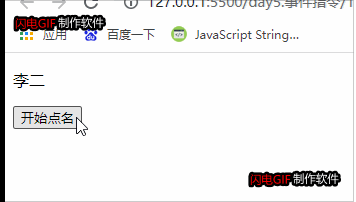﻿ Vue做一个简单的随机点名册_vue.js_脚本之家
vue.jsVue做一个简单的随机点名册

<div id="app">
<p>{{result}}</p>
<button @click="randomName()">{{txt}}</button>
</div>

Vue部分：

<script>
let vm = new Vue({
el:'#app',
data:{
list:["小一","李二","王三","周四","张五"],
// 随机点名的内容
result:'',
// 按钮文本内容
txt:"开始点名",
// 流程控制开关
open:true,
// 定义计时器开关
timer:null
},
methods: {
move(){
// 获取一个 0-当前数组长度的随机数
let random = Math.floor(Math.random()*(this.list.length-0))

// 让随机数成为 list数组的随机下标，赋值给 result ，在页面渲染
this.result = this.list[random]
},
randomName(){
// 流程控制开关
if(this.open){
// 定义计时器，调用move方法
this.timer = setInterval(this.move,100)
this.txt = "停止点名"
this.open = false
}else{
// 清除计时器
clearInterval(this.timer)
this.txt = "开始点名"
this.open = true
}
}
}
})
</script>